Next: A quantum mechanics example Up: Examples of applications of Previous: Robot workspace analysis   Contents

Robot synthesis example

The robot presented in the previous sections has numerous advantages but its performances are very sensitive to its geometry (i.e. to the location of the points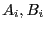). The previous sections have presented analysis problems: analyze for a given robot what are its performance. But we may also consider an even more complex problem which is the synthesis problem i.e. find what should be the geometry of the robot such that it satisfies some performance criteria. This is very complex issue but one which has clearly a large impact in practice (for example when designing a robot whose load will be over 2 tons and whose accuracy should be better than a micrometer as considered by the European Synchrotron Radiation Facility in Grenoble).

Consider for example the following problem: what should be the geometry of the robot (i.e. the location of theand the limits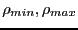on the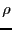) such that a given set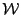of position/orientation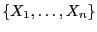can be reached by the platform ?

The inverse kinematic analysis allows to obtain the constraints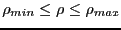as a set of inequalities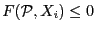between the design parameters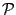and the elements of the set. A classical approach to solve this problem will be to determine the setthat minimize the cost function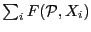. But this approach has many drawbacks:

• we cannot guarantee to find even one solution
• it will give at most a few solutions to the synthesis problem while in general there will be an infinite number of solutions
• for a given solution the geometry of the real robot will be different from the theoretical solution due to the manufacturing tolerances. Hence we cannot guarantee that the real robot will really be able to reach the setInterval analysis is a more appropriate approach. First of all it is reasonable to assume that the geometrical parameters are bounded as they define the overall size of the robot. We may thus define a box as a set of ranges, one for each design parameters. Using interval arithmetics we are then able to compute the lower and upper bounds of each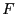for each element of. The different cases that may occur for a box are:
• the lower bound of the interval for oneis positive: we discard the box
• the upper bound of the intervals for allis negative: the box is stored as a solution
• the lower bound of the intervals for allis negative while their upper bound is positive: we bisect the box at the variable having the largest range except if all ranges have a width that is lower or equal to twice the manufacturing errors, in which case we discard the box

Using this approach we get not only one solution but ranges for the solution: indeed each point of the solution boxes satisfy all inequalitieswhich means that the theoretical robot will be able to reach all the elements of. But we may take also the manufacturing errors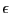into account. Indeed for a range A=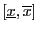in a solution box we may consider the range AP=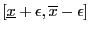that is included in the previous range and exists as the ranges of a solution box have a width at least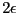. If we choose as design parameter a point in AP, then for the real robot the design parameter value will be included in A. As this is true for all the design parameters, then we may guarantee that the real robot will be able to reach all elements of.

Using this approach we are able to find all the geometries such that the real robot satisfy the workspace constraints. Now we may consider another design criteria and use the same approach, that will lead to another set of design parameters. Taking the intersection of this set with the set obtained for the workspace we will be able to compute all robot geometries that satisfy the new design criteria and the workspace constraint.

Here again interval analysis is an elegant approach that allows to solve a very practical problem. This approach has been used by the COPRIN project in various industrial contracts.Next: A quantum mechanics example Up: Examples of applications of Previous: Robot workspace analysis   Contents
Jean-Pierre Merlet 2012-12-20